Next: Results Up: enam04piotr-07w Previous: Introduction

# Method

By using the code HFBTHO , we perform the HFB calculations within the particle space of 20 harmonic oscillator shells, which leads to the single-particle energies of 200MeV and above. When no truncation is performed in the quasiparticle space, the Bogoliubov transformation is unitary, and this guarantees that the pairing tensor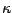is antisymmetric. This is no longer true for the finite energy cutoff. The inset in Fig. 1 shows the maximum matrix element of the symmetric and antisymmetric parts of the pairing tensor as functions of the cutoff energy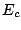in the quasiparticle space. Typically, the former does not exceed 1% of the latter; however, a non-zero symmetric component means that the fermion quasiparticle state representing the HFB ground state does not exist. Usually one simply disregards this symmetric part in the Skyrme-HFB calculations.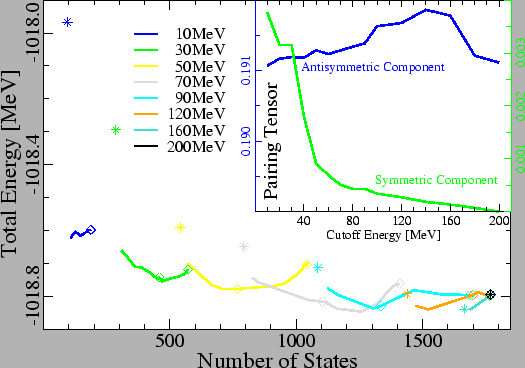Our method ensures the antisymmetricity of the pairing tensor and, at the same time, keeps the number of quasiparticle states limited. The approach is based on finding an optimal truncated particle space, dictated by a given quasiparticle truncation, in which the HFB equations are solved without any further cutoff. Full-space diagonalization of the HFB equations is necessary only to provide the aforementioned optimal basis.

The Singular Value Decomposition (SVD)  is an algebraic method, which, by means of finding the so-called singular values, orders orthonormal basis states according to their importance for decomposition of a rectangular matrix into a sum of components. We use it to decompose the combined matrix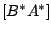, where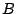and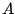are the Bogoliubov matrices corresponding to a non-unitary transformation, and assemble the optimal basis by taking only those particle states which have the corresponding singular values above a certain SVD cutoff,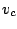. Since for each value of the SVD cutoff the dimension of the resulting particle space is different, one has to refit the pairing strength as function of. We do it so that the pairing gap is the same in both steps: in the full-space solution obtained for a given energy cutoff and in the truncated-space solution. Our calculations are carried out according to the following scheme:

1. Self-consistent solution of the HFB equations in the full space.

2. Singular Value Decomposition of the combined matrixcorresponding to the full-space solution.

3. Defining the truncated particle space by keeping the SVD states that correspond to singular values above the SVD cutoff.

4. Self-consistent solution of the HFB equations in the truncated particle space.

5. Fitting the pairing strength by repeating step (d) with different pairing strengths until the pairing gaps in the full and truncated spaces are equal.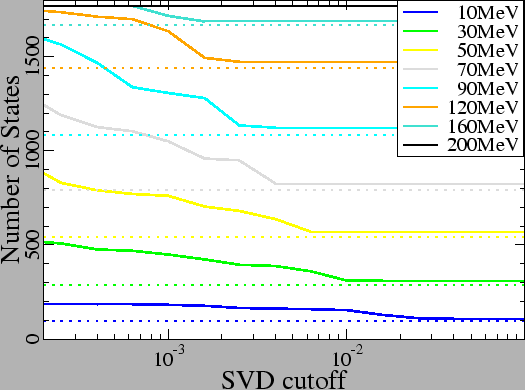Next: Results Up: enam04piotr-07w Previous: Introduction
Jacek Dobaczewski 2005-01-23